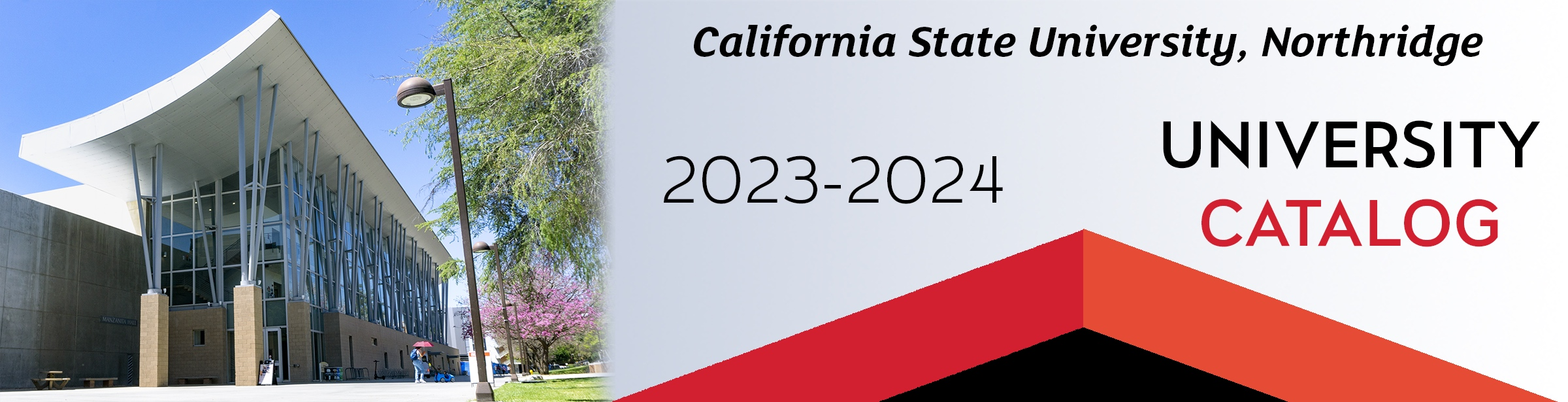## Course: MATH 262. Introduction to Linear Algebra (3)

Prerequisite: Completion of MATH 150B with a grade of “C” or better. Systems of linear equations, matrices, determinants, eigenvalues, vector spaces and linear transformations, as well as introduction to inner products on Rn and spectral theorem for symmetric matrices.

### MATH 262

Class NumberLocationDayTime